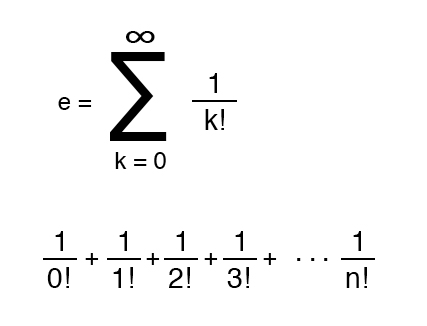Vol. EE Reference
Chapter 4 Algebra Reference

# Important Constants

### Euler’s Number

Euler’s constant is an important value for exponential functions, especially scientific applications involving decay (such as the decay of a radioactive substance).

It is especially important in calculus due to its uniquely self-similar properties of integration and differentiation.

e approximately equals:
2.71828 18284 59045 23536 02874 71352 66249 77572 47093 69996### Pi

Pi (π) is defined as the ratio of a circle’s circumference to its diameter.

Pi approximately equals:
3.14159 26535 89793 23846 26433 83279 50288 41971 69399 37511


Note:

For both Euler’s constant (e) and pi (π), the spaces shown between each set of five digits have no mathematical significance.

They are placed there just to make it easier for your eyes to “piece” the number into five-digit groups when manually copying.

RELATED WORKSHEETS:

#### Lessons in Electric Circuits

##### Volumes »
Published under the terms and conditions of the Design Science License